# Samacheer Kalvi 6th Maths Solutions Term 3 Chapter 5 Information Processing Ex 5.1## Tamilnadu Samacheer Kalvi 6th Maths Solutions Term 3 Chapter 5 Information Processing Ex 5.1

Question 1.
Study and complete the following pattern.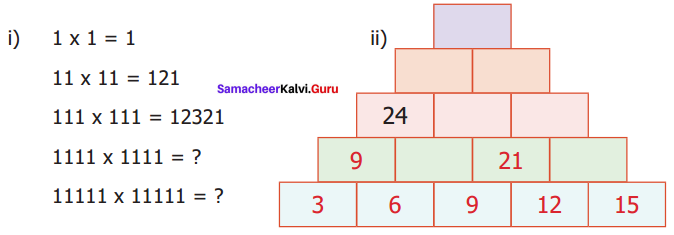Solution: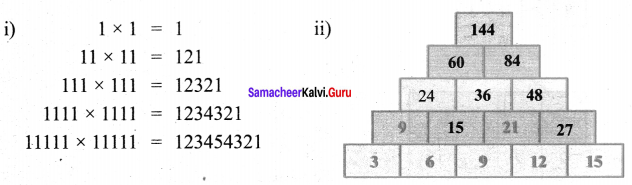Question 2.
Find next three numbers in the following number patterns,
i) 50, 51, 53, 56, 60,…
ii) 77, 69, 61, 53,…
iii) 10, 20, 40, 80, …..
iv) $$\frac{21}{33}, \frac{321}{444}, \frac{4321}{5555}$$
Solution:
i) The pattern generating these numbers is
50, 50 + 1, 51 + 2, 53 + 3, 56 + 4, 60 + 5, 65 + 6, 71 + 7,
∴ 50, 51, 53, 56, 60, 65, 71, 78, ……
∴ The next three numbers will be 65, 71, 78

ii) The pattern generating these numbers is
77, 77 – 8, 69 – 8, 61 – 8, 53 – 8, 45 – 8, 37 – 8, 29
77, 69, 61, 53, 45, 37, 29, 21,
∴ The next three numbers will be 45, 37, 29.

iii) The pattern generating these numbers is
10, 10 + 10, 20 + 20, 40 + 40, 80 + 80, 160 + 160, 320 + 320,….
10, 20, 40, 80, 160, 320, 640,….
∴ The next three numbers will be 160, 320, 640.

iv) The pattern generating these numbers is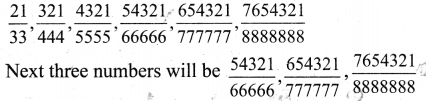Question 3.
Consider the Fibonacci sequence 1, 1, 2, 3, 5, 8, 13, 21, 34, 55,… Observe and complete the following table by understanding the number pattern followed. After filling the table discuss the pattern followed in addition and subtraction of the numbers of the sequence.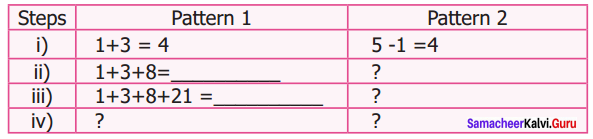Solution: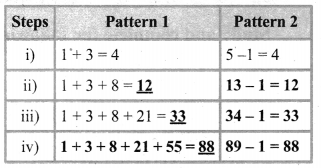Question 4.
Complete the following patterns.Solution: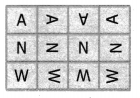Rotate the figure 90°C clockwise to get the next figure.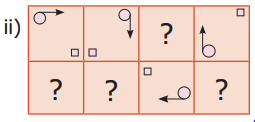Solution: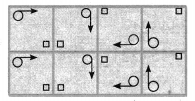* Move the circled arrow to each comer clockwise and place the square to the opposite comer of the arrow, in first row.
* Copy the first row in the second row.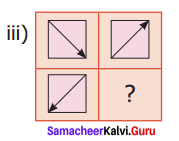Solution: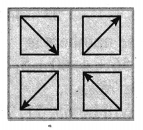Rotate the arrow inside the square 90° anticlockwise direction so that the arrow pointing each comer anticlockwise.Question 5.
Find HCF of the following pair of numbers by Euclid’s game.
(i) 25 and 35
(ii) 36 and 12
(iii) 15 and 29
Solution:
(i) HCF of (25, 35 – 25)
25 = 5 × 5
10 = 2 × 5
HCF of (25, 10) = 5

(ii) HCF of (36, 36 – 12)
36 = 2 × 2 × 3 × 3
24 = 2 × 2 × 2 × 3
HCF of (36, 24) = 2 × 2 × 3 = 12

(iii) HCF of (15, 29 -15)
15 = 3 × 5 × 1
14 = 2 × 7 × 1
HCF of (15, 14) = 1

Question 6.
Find HCF of 48 and 28. Also find the HCF of 48 and the number Obtained by finding their difference.
Solution: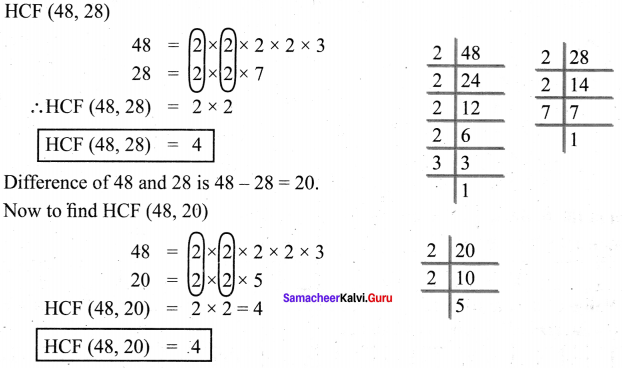Question 7.
Give instructions to fill in a bank withdrawal form issued in a bank.
Solution:

• The name should be written in capital letters from left to right.
• Write the date of withdrawal on the right top comer of the form.
• Write the amount (in words) to be withdrawn in the space provided.
• Write the amount (in figures) to be withdrawn in the box provided.
• Put your signature at the right bottom above the ‘signature of the depositor’.Question 8.
Arrange the name of your classmates alphabetically.
Solution:
Name of my classmates is given below.

1. Akila
2. Akshaya
3. Bharathi
4. Divya
5. Ezhil
6. Fathima
7. Gayathri
8. Hlelen
9. Irusammal
10. Joy
11. Kaviya
12. Lakshmi
13. Monika
14. Nisha
15. Olin
16. Patsy
17. Queenlin
18. Ratha
19. Sindhu
20. Vidhya

Question 9.
Follow and execute the instructions given below?
i) Write the number 10 in the place common to the three figures
ii) Write the number 5 in the place common for square and circle only.
iii) Write the number 7 in the place common for triangle and circle only.
iv) Write the number 2 in the place common for triangle and square only.
v) Write the numbers 12,14 and 8 only in square, circle and triangle respectively.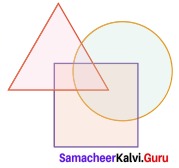Solution: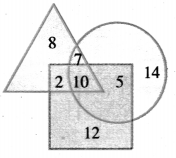Question 10.
Fill in the following information.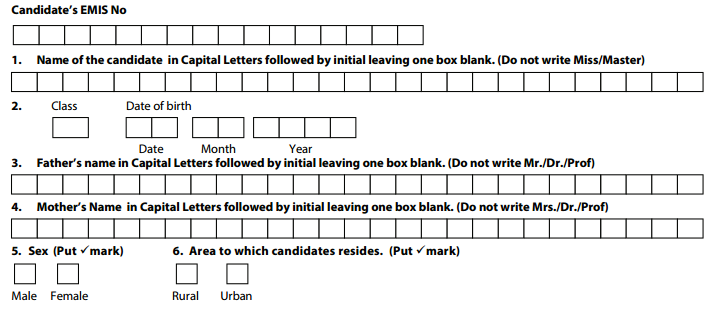Solution: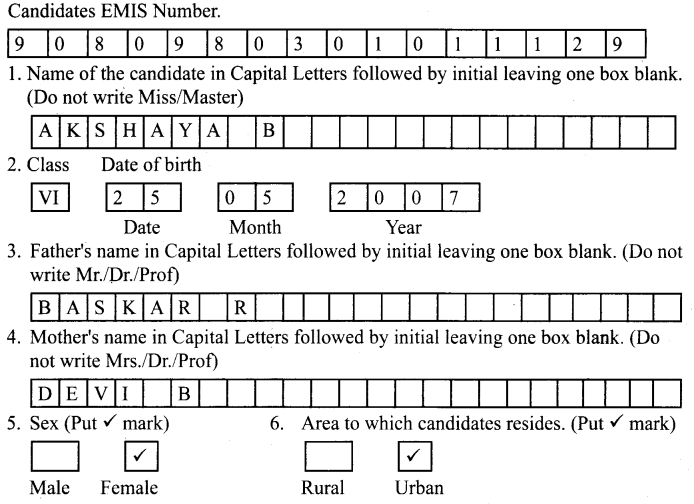Objective Type Questions

Question 11.
The next term in the sequence 15, 17, 20, 22, 25, is
(a) 28
(b) 29
(c) 27
(d) 26
Solution:
(c) 27

Question 12.
What will be the 25th letter in the pattern? ABCAABBCCAAABBBCCC
(a) B
(b) C
(c) D
(d) A
Hint:
Write A, B, C with increasing number of A, B, and C
Solution:
(a) B

Question 13.
The difference between 6th term and 5th term in the Fibonacci sequence is
(a) 6
(b) 8
(c) 5
(d) 3
Solution:
(d) 3

Question 14.
The 11th term in the Lucas sequence 1, 3, 4, 7,… is
(a) 199
(b) 76
(c) 123
(d) 47
Solution:
(a) 199

Question 15.
If the Highest Common Factor of 26 and 54 is 2, then HCF of 54 and 28 is
(a) 26
(b) 2
(c) 54
(d) 1
Solution:
(b) 2